2012/12/08 11:35

绘图手册

Created Friday 07 December 2012

matplotlib.pyplot是一系列的命令风格的函数，让matplotlib工作起来像MATLAB。每个pyplot函数都对视图窗口起着作用。例如，新建一个视图窗口，在视图窗口中新建一个绘图区域，在其中绘制一些线条，或者用标签装饰绘图等等......matplotlib.pyploti是保持状态的，所以它一直跟踪着当前视图窗口和绘图区域，以及绘图函数可以直接作用到当前坐标轴。

import matplotlib.pyplot as plt
plt.plot([1, 2, 3, 4])
plt.ylabel('some numbers')
plt.show()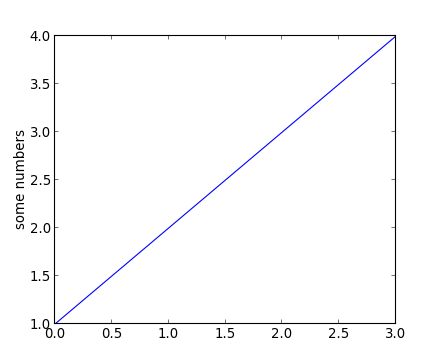plot()是一个万能的命令，可以接收任意数量的参数，例如，绘制x轴对y轴，你可以使用命令：
plt.plot([1,2,3,4], [1,4,9,16])

import matplotlib.pyplot as plt
plt.plot([1,2,3,4], [1,4,9,16], 'ro')
plt.axis([0, 6, 0, 20])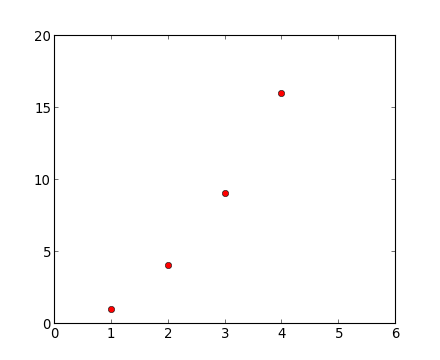查看plot()文档了解所有的线型以及格式字符串。在上面例子中axis()命令的参数是[x轴最小值，x轴最大值，y轴最小值，y轴最大值]的列表，它指定了坐标轴的视点。

import numpy as np
import matplotlib.pyplot as plt
t = np.arange(0, 5, 0.2)
plt.plot(t, t, 'r--', t, t2, 'bs', t, t3, 'g^')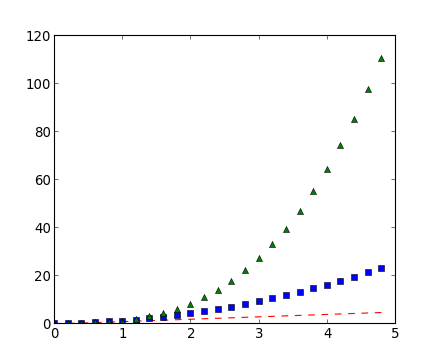3.1 控制线条属性

· 使用关键字参数：

plt.plot(x, y, linewidth=2.0)

· 使用Line2D的实例的setter方法。

plot返回一个线条列表，如line1, line2 = plot(x1, y1, x2, y2).下面只有一条线条，所以这个线条列表的长度为1。 我使用元组解包(unpacking) line, = plot(x, y, 'o')来获得列表的第一个元素。
line, = plt.plot(x, y, '-')
line.set_antialiased(False) # 关闭抗锯齿

· 使用setp()命令

lines = plt.plot(x1, y1, x2, y2)
# 使用关键字参数
plt.setp(lines, color='r', linewidth=2.0)
# 使用MATLAB风格的字符串/值对
plt.setp(lines, 'color', 'r', 'linewidth', 2.0)

Line2D属性的可选项：

alpha float
animated [True | False]
antialiased or aa [True | False]
clip_box 一个matplotlib.transform.Bbox实例
clip_on [True | False]
clip_path 一个Path实例和一个Transform实例, a Patch
color or c any matplotlib color
contains the hit testing function
dash_capstyle [‘butt’ | ‘round’ | ‘projecting’]
dash_joinstyle [‘miter’ | ‘round’ | ‘bevel’]
dashes sequence of on/off ink in points
data (np.array xdata, np.array ydata)
figure 一个matplotlib.figure.Figure实例
label 任何字符串
linestyle or ls [ ‘-‘ | ‘–’ | ‘-.’ | ‘:’ | ‘steps’ | ...]
linewidth or lw float value in points
lod [True | False]
marker [ ‘+’ | ‘,’ | ‘.’ | ‘1’ | ‘2’ | ‘3’ | ‘4’
markeredgecolor or mec any matplotlib color
markeredgewidth or mew float value in points
markerfacecolor or mfc any matplotlib color
markersize or ms float
markevery None | integer | (startind, stride)
picker 使用在交互式环境中的线条选择
solid_capstyle [‘butt’ | ‘round’ | ‘projecting’]
solid_joinstyle [‘miter’ | ‘round’ | ‘bevel’]
transform 一个matplotlib.transforms.Transform实例
visible [True | False]
xdata np.array
ydata np.array
zorder 任何数字

In : lines = plt.plot([1,2,3])
In : plt.setp(lines)

alpha: float
animated: [True | False]
antialiased or aa: [True | False]
...snip

3.2 使用多视图窗口和坐标轴

MATLAB和pyplot，都有当前视图窗口和当前坐标轴的概念。所有的绘图命令都会作用到当前的坐标轴。gca()函数返回当前的坐标轴(一个matplotlib.axes.Axes的实例)，gcf()函数返回当前视图窗口(一个matplotlib.figure.Figure实例)。一般来说，你不用为这个担心，因为它会对后面的情形操心的。下面的小程序将会新建两个子视图：

import numpy as np
import matplotlib.pyplot as plt

def f(t):
return np.exp(-t) * np.cos(2*np.pi*t)
t1 = np.arange(0, 5, 0.1)
t2 = np.arange(0, 5, 0.02)
plt.figure(1)
plt.subplot(211)
plt.plot(t1, f(t1), 'bo', t2, f(t2), 'k')

plt.subplot(212)
plt.plot(t2, np.cos(2*np.pi*t2), 'r--')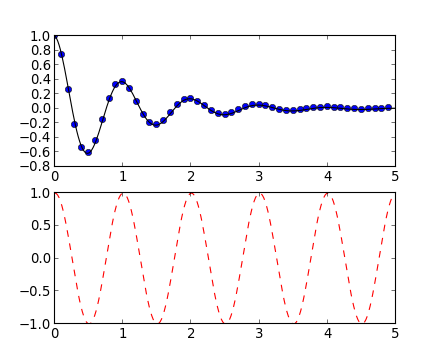import matplotlib.pyplot as plt
# 第一个视图窗口
plt.figure(1)
# 第一个视图窗口中的第一个子视图
plt.subplot(211)
plt.plot([1,2,3])
# 地一个视图窗口中的第二个子视图
plt.subplot(212)
plt.plot([4,5,6])

# 第二个视图窗口
plt.figure(2)
# 默认创建subplot(111)
plt.plot([4,5,6])
# 切换到第一个视图窗口，并且当前的子视图还是subplot(212)
plt.figure(1)
# 使地一个视图窗口的subplot(211)及第一个子视图为当前子视图
plt.subplot(211)
# 第一个子视图的标题
plt.title('Easy as 1,2,3')

3.3 使用文本

text()命令可以在任何位置添加文本，xlabel()、ylabel()和title()用来在指定的位置上添加文本。(获得更多详细例子请查看文本简介)

import numpy as np
import matplotlib.pyplot as plt

mu, sigma = 100, 15
x = mu + sigma * np.random.randn(10000)
# 数据直方图
n, bins, patches = plt.hist(x, 50, normed=1, facecolor='g', alpha=0.75)
plt.xlabel('Smarts')
plt.ylabel('Probability')
plt.title('Histogram of IQ')
plt.text(60, 0.25, r'$\mu=100, \ \sigma=15$')
plt.axis([40, 160, 0, 0.03])
plt.grid(True)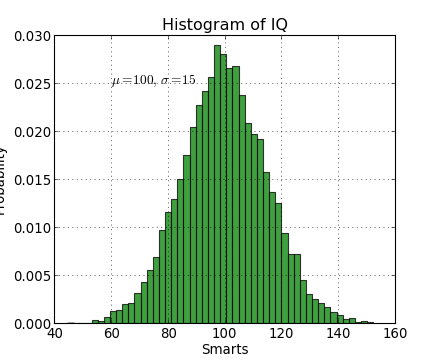t = plt.xlabel('my data', fontsize=14, color='red')

3.3.2 注释文本

import numpy as np
import matplotlib.pyplot as plt
ax = plt.subplot(111)

t = np.arange(0, 5, 0.01)
s = np.cos(2*np.pi*t)
line, = plt.plot(t, s, lw=2)
plt.annotate('local max', xy=(2,1), xytext=(3,1.5), arrowprops=dict(facecolor='black', shrink=0.05),)
plt.ylim(-2,2)
plt.show()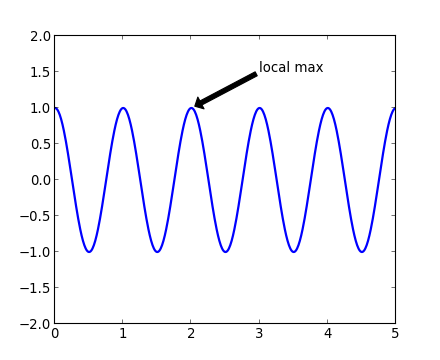在这个简单的例子中，xy（箭头）和xytext位置（文本位置）都在数据范围中的坐标。

1
2 收藏

作者的其它热门文章import numpy as np
import matplotlib.pyplot as plt
t = np.arange(0, 5, 0.2)
plt.plot(t, t, 'r--', t, t**2, 'bs', t, t**3, 'g^')
2016/01/30 16:40

1 评论
2 收藏
1